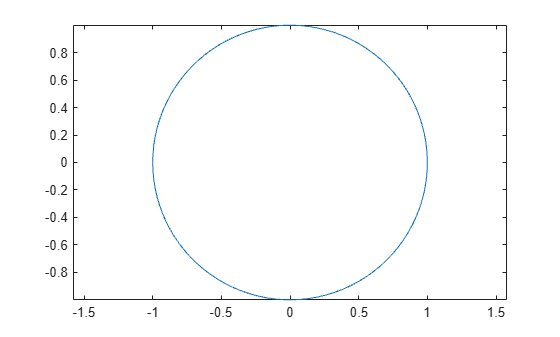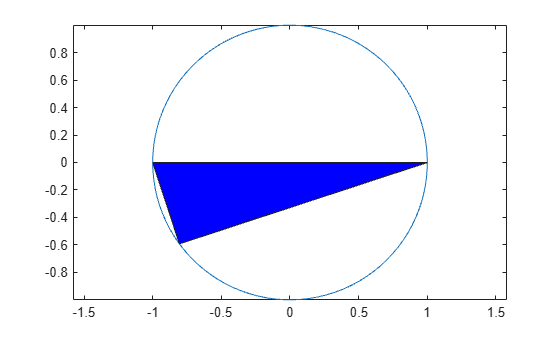# Animate Graphics Object

This example shows how to animate a triangle looping around the inside of a circle by updating the data properties of the triangle.

Plot the circle and set the axis limits so that the data units are the same in both directions.

```theta = linspace(-pi,pi); xc = cos(theta); yc = -sin(theta); plot(xc,yc); axis equal ```Use the `area` function to draw a flat triangle. Then, change the value of one of the triangle vertices using the (x,y) coordinates of the circle. Change the value in a loop to create an animation. Use a `drawnow` or `drawnow limitrate` command to display the updates after each iteration. `drawnow limitrate` is fastest, but it might not draw every frame on the screen.

```xt = [-1 0 1 -1]; yt = [0 0 0 0]; hold on t = area(xt,yt); % initial flat triangle hold off for j = 1:length(theta)-10 xt(2) = xc(j); % determine new vertex value yt(2) = yc(j); t.XData = xt; % update data properties t.YData = yt; drawnow limitrate % display updates end ```The animation shows the triangle looping around the inside of the circle.